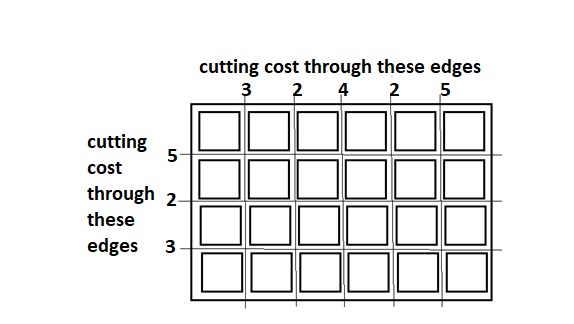# Minimum Cost to cut a board into squares in Python

Suppose we have a board of length p and width q; we have to break this board into p*q number of squares such that cost of breaking is as minimum as possible. Cutting cost for each edge will be given.

So, if the input is like X_slice = [3,2,4,2,5], Y_slice = [5,2,3]then the output will be 65

To solve this, we will follow these steps −

• res := 0
• horizontal := 1, vertical := 1
• i := 0, j := 0
• while i < m and j < n, do
• if X_slice[i] > Y_slice[j], then
• res := res + X_slice[i] * vertical
• horizontal := horizontal + 1
• i := i + 1
• otherwise,
• res := res + Y_slice[j] * horizontal
• vertical := vertical + 1
• j := j + 1
• total := 0
• while i < m, do
• total := total + X_slice[i]
• i := i + 1
• res := res + total * vertical
• total := 0
• while j < n, do
• total := total + Y_slice[j]
• j := j + 1
• res := res + total * horizontal
• return res

## Example

Let us see the following implementation to get better understanding −

Live Demo

def minCost(X_slice, Y_slice, m, n):
res = 0
X_slice.sort(reverse = True)
Y_slice.sort(reverse = True)
horizontal = 1
vertical = 1
i = 0
j = 0
while i < m and j < n:
if (X_slice[i] > Y_slice[j]):
res += X_slice[i] * vertical
horizontal += 1
i += 1
else:
res += Y_slice[j] * horizontal
vertical += 1
j += 1
total = 0
while (i < m):
total += X_slice[i]
i += 1
res += total * vertical
total = 0
while (j < n):
total += Y_slice[j]
j += 1
res += total * horizontal
return res
m = 6; n = 4
X_slice = [3,2,4,2,5]
Y_slice = [5,2,3]
print(minCost(X_slice, Y_slice, m-1, n-1))

## Input

[3,2,4,2,5],[5,2,3]

## Output

65